# Ea-467 attitude control lab introduction

 Date 23.04.2018 Size 34.19 Kb. #46091
EA-467 ATTITUDE CONTROL LAB Introduction (rev-b) Fall 2017
Reversed order. Now do physical measurements first. Theoretical second

This lab is the first in a three lab series for Attitude Determination and Control (ADCS). The objective is to build your LABsat so that it can de-spin itself and then point its solar panel at the Sun. Your first lab will determine the physical properties of your spacecraft (moments of inertia and center of gravity). The next lab will develop your Sun sensor and magnetometer for attitude determination or estimation and then the third lab will develop your control system using active magnetic stabilization, thrusters and possibly momentum wheels. The forces needed to change the attitude of a spacecraft are extremely small in space, but are effective because all other forces acting on the spacecraft in the vacuum and microgravity of space are even smaller. In the lab environment, we can minimize any friction in one axis by hanging our models on a thin string and can use these models to see the principles involved in 4 out of 5 of the below-listed attitude control methods.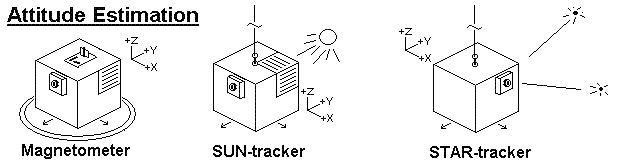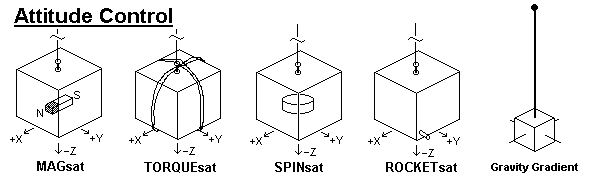Part A: Measure the Center of Mass: Find the center of mass of your LAB sat by using a knife-edge in the three axes. To measure the X and Y axis CM, you can balance the LABsat upright on a knife-edge (piece of aluminum angle). Place the far side edge right on the center mark of the battery tray. Then lift and adjust the front only. When you find the balance point, the near-side offset needed to find balance is twice the actual offset in the middle (triangle geometry rule). For the Z offset, use the small wire-hanger to suspend your satellite along the X axis. Carefully align the satellite perfectly horizontal in that axis so you can measure the Z dimension of that hanging point as observed on the corner posts. Measure from the bottom of the battery board. Compare your measured COM to that calculated in part C.
##### Part B: Measuring your spacecraft’s Moment of Inertia: For computing spacecraft dynamics, you need to know the moment of inertia of your spacecraft. You will first measure it empirically, and then post-lab, calculate it for verification.

T = K * θ, and P is used to calculate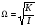, where Ω = 2 π f and f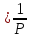To make the measurement, place the 862g brass cylinder on the string without imparting any twists. Then wind it up 3 turns and gently release it. Observe the angular half periods of oscillation (it is only a half-period from stop-to-stop). Use the period, the mass and the calculated MOI for this standard cylinder, to derive the string constant K.

##### Now that you have computed the string’s spring constant, you can use that knowledge to measure the moment of inertia of your spacecraft. Hang your LABsat on its Z axis harness, wind the string 3 turns and then gently release it (with out imparting any torque). Let it oscillate back and forth and observe three half-periods. Using the average period and the string’s spring constant, you can compute the spacecraft’s observed Z axis MOI. For the other two axes, use the special wire-hanger you used in part C to hang the satellite in the other two axes and repeat the MOI half-period measurements for X and Y. Post lab, you will compare your measured moment of inertia (about X, Y, and Z) with the computed values from Part C. Comment on possible reasons for any discrepancies. You will use the LABsat moment of inertia in later parts of this ADCS lab series.

Part C: Predicting the Moment of Inertia: The moment of inertia about an axis is simply the sum of the moments of inertia of all the components of a spacecraft about that axis. Apply the parallel axis theorem to any masses that are off-center. Use a scale and ruler to measure the mass and location of all components of your LABsats. The origin of the structural coordinate system is the bottom center of the battery tray with +Z up. You are given a mass table in Excel so you can sum the results and find the spacecraft CM which will be the origin of the Body coordinate frame. The inertia of the entire vehicle is the sum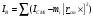where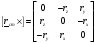Where ICMi is the inertia of the component about its own center of mass, mi.

Note, add 10g for each yellow NiCd cell in your battery tray they are heavier than the green ones.

Let a,b, and c represent the dimensions of each component along the x, y and z axes, respectively. Let Sx,Sy,Sz be the distances of the components center to the structural frame origin, and rcm (made up of rx, ry, and rz) is the vector from the vehicle center of mass to the component center of mass. Record data in columns such as |m| a,b,c | then compute the individual ICMi (i.e. ICMx, ICMy, ICMz) for each object. Then record the component location |Sx,Sy,Sz| in the structural coordinate system and then compute the overall center of mass as CMx,CMy,CMz. This is the origin of the LABsat body frame. Next, continue the table by computing the offset |rx,ry,rz| of each component to the origin of the body frame (spacecraft center of mass) and finally use the parallel axis theorem to compute |Ix,Iy,Iz| for each component. Add up the component moments of inertia to compute the overall |Ix,Iy,Iz| for the LABsat.

In Part A you measured your center of mass (COM) experimentally and in Part B you measured the moments of intertia (MOI) experimentally for comparison to the calculated MOI and COM you calculate in this part C.